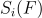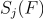# ACI: Adjacent-channel interference

Is there any information about the influence of Wi-Fi channels on each other?

The most reasonable approach seems to be published in this article

Mi, Penghui, and Xianbin Wang. "Improved channel assignment for WLANs by exploiting partially overlapped channels with novel CIR-based user number estimation"

It consists in the following:

1. The coefficient of influence of one channel on another for OFDM signals can be calculated using the formula:where i and j are the channel numbers,is the distance between the central frequencies of the channels,and- distributions of signal power over the frequency range of channels i and j, respectively. These distributions are determined by the spectral masks of the OFDM signals (Fig. 1).The authors define this parameter as the I-factor and provide the normalized results of its calculation in the following graph (Fig. 2).2. This parameter has a cumulative effect.

To calculate the quality of channels, the authors propose the term equivalent number of users on a channel:where C is the set of 802.11 channels, and N(j) is the number of users on a particular channel. Accordingly, the channel with the smallest equivalent number of users will be considered the best channel.

The results shown in the figure 2 are refined based on the results of experiments. However, we should mention that these indicators are relevant for the case when both the interfering and the device in question operate with the same bandwidth spectrum of 20 MHz. However, the 802.11 n standard assumes the possibility of using both 20 and 40 MHz (channel bonding). The following publication provides spectral masks for 40 MHz wide signals:

V. Shrivastava, S. Rayanchu, J. Yoon, and S. Banerjee, “802.11n under the microscope”, Internet Measurement Conference ’08, 2008.Using these spectral characteristics and the same method as described in formula (1), the authors obtain the interference factors for four different cases:

• interfering device and device in question use signals with a 20 MHz bandwidth (20T-20R)
• both use 40 MHz (40T-40R)
• interfering - 40 MHz, device in question - 20 MHz (40T-20R); interfering 20 MHz, device in question - 40 MHz (20T-40R)Provided that we are only interested in cases where the device in question occupies 20 MHz (optimal for the 2.4 GHz spectrum), the results can be shown in the following table:We should mention that the expansion of the spectrum does not occur symmetrically, but either to the left or to the right side. For example, if the device was on channel 10 at 20 MHz, then in the case of 40 MHz, the channel 10 will be primary, and the channel 6 secondary. In this case, there are the following rules for resolution collisions during the multiple access stage: the primary channel is considered busy if it detects a signal that exceeds the CS (carrier sence) threshold; the secondary channel is considered busy if a signal is detected either on the primary or secondary channel, or on both channels (see 802.11 n 20/40 MHz BSS Mode Rules). Therefore, it is not possible to consider the mechanism of expanding the spectrum as a mechanism for adding an independent device to the spectrum, by means of which the part of the information will be transmitted. That is why it is proposed to review the extended part of the spectrum by considering it as an ACI.

## Cloud ACS (CWMP/TR-069, USP/TR-369)

Cloud service for device management (“Cloud DMS”) is a TR-069 ACS and TR-369 Controller for remote provisioning, management and monitoring all kind of devices that support CWMP/USP protocols.